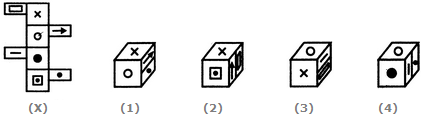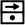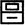# Non Verbal Reasoning - Cubes and Dice - Discussion

### Discussion :: Cubes and Dice - Section 1 (Q.No.23)

The sheet of paper shown in the figure (X) given on the left hand side, in each problem, is folded to form a box. Choose from amongst the alternatives (1), (2), (3) and (4), the boxes that are similar to the box that will be formed.

23.

Choose the box that is similar to the box formed from the given sheet of paper (X).[A]. 1 and 2 only [B]. 1 and 3 only [C]. 3 and 4 only [D]. 1, 2, 3 and 4

Answer: Option B

Explanation:

The fig. (X) is similar to the Form VIII. So, when a cube is formed by folding the sheet shown in fig. (X), thenandare the two faces and these two faces lie opposite to each other. Also, the face bearing the 'x' sigh lies opposite to the face bearing the black circle and the face bearing the white circle lies opposite to the face bearing the square (having a dot inside it). Now, the cubes in figures (2) and (4) consist of faces which are not formed when the sheet in fig. (X) is folded. Hence, these two cubes are not, formed. Therefore, only the cubes in figures (1) and (3) are formed.

 Preethi said: (Sep 10, 2016) Nice explanation.

#### Post your comments here:

Name *:

Email   : (optional)

» Your comments will be displayed only after manual approval.

#### Current Affairs 2021

Interview Questions and Answers